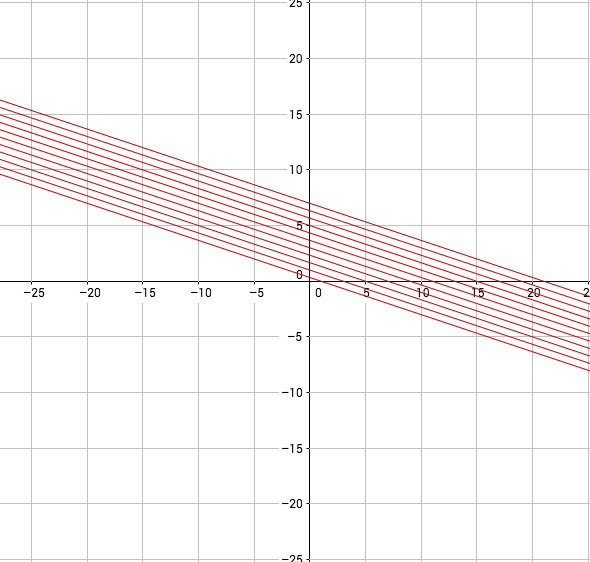# Linear Equations in Two Variables

## Q1

A test has 25 questions for a total score of 150 points. Among the 25 questions, each multiple choice question carries 3 points and the descriptive type question question carries 8 points. How many multiple choice questions and descriptive type questions are there in the test?

Express the above problem as a pair of linear equations in two variables. State what the assumed variables represent.

## Plot the two linear equations in the applet

How many multiple choice questions are there in the quiz?

How many descriptive questions are there in the quiz?

## Create Patterns with LinesEquation of one of the lines in above pattern is Can you re-create this pattern in the applet given below?

## Complete the rectangle

In the following applet , draw two straight lines such that the points of intersection of the lines form a rectangle with sides of length 6 units and 8 units. A is one of the vertices of this rectangle. Find the points of intersection of the lines and Label the points.

## Vertices of Rectangle

What are the three vertices of the rectangle?